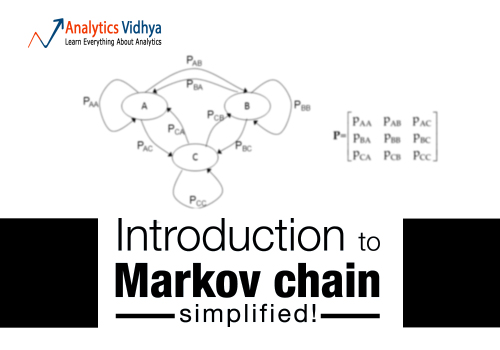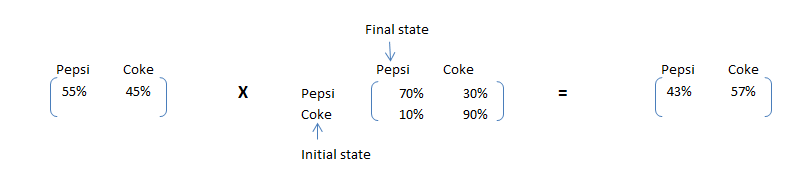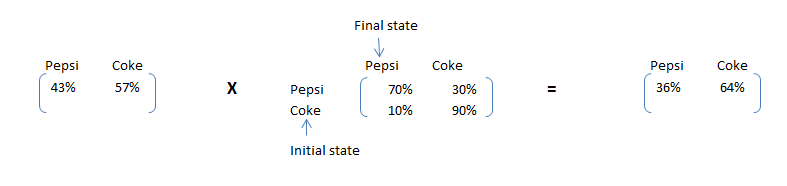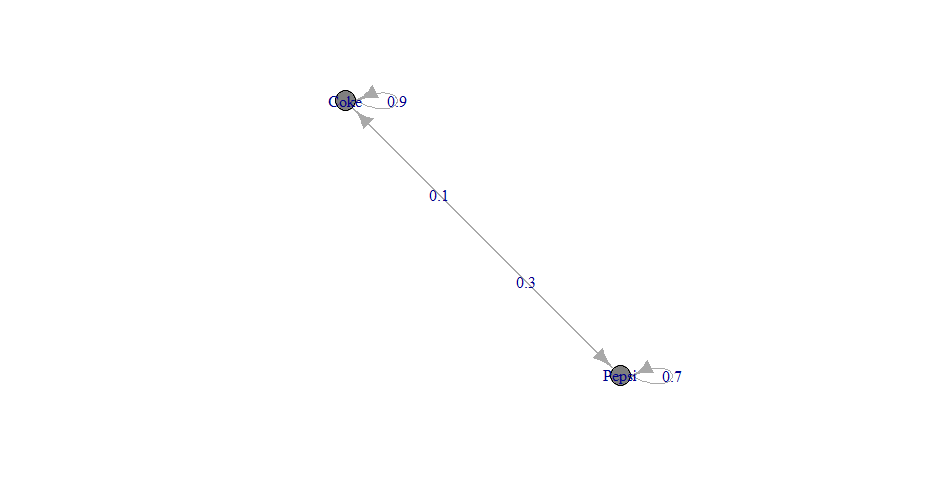Home » Introduction to Markov chain : simplified! (with Implementation in R)

# Introduction to Markov chain : simplified! (with Implementation in R)

Markov chain is a simple concept which can explain most complicated real time processes.Speech recognition, Text identifiers, Path recognition and many other Artificial intelligence tools use this simple principle called Markov chain in some form. In this article we will illustrate how easy it is to understand this concept  and will implement it in R.Markov chain is based on a principle of “memorylessness”. In other words the next state of the process only depends on the previous state and not the sequence of states. This simple assumption makes the calculation of conditional probability easy and enables this algorithm to be applied in number of scenarios. In this article we will restrict ourself to simple Markov chain. In real life problems we generally use Latent Markov model, which is a much evolved version of Markov chain. We will also talk about a simple application of Markov chain in the next article.

Coke and Pepsi are the only companies in country X. A soda company wants to tie up with one of these competitor. They hire a market research company to find which of the brand will have a higher market share after 1 month. Currently, Pepsi owns 55% and Coke owns 45% of market share. Following are the conclusions drawn out by the market research company:

P(P->P) : Probability of a customer staying with the brand Pepsi over a month = 0.7

P(P->C) : Probability of a customer switching from Pepsi to Coke over a month = 0.3

P(C->C) : Probability of a customer staying with the brand Coke over a month = 0.9

P(C->P) : Probability of a customer switching from Coke to Pepsi over a month = 0.1

We can clearly see customer tend to stick with Coke but Coke currently has a lower wallet share. Hence, we cannot be sure on the recommendation without making some transition calculations.

Transition diagram

The four statements made by the research company can be structured in a simple transition diagram.The diagram simply shows the transitions and the current market share. Now, if we want to calculate the market share after a month, we need to do following calculations :

Market share (t+1) of Pepsi = Current market Share of Pepsi * P(P->P) + Current market Share of Coke * P(C->P)

Market share (t+1) of Coke = Current market Share of Coke * P(C->C) + Current market Share of Pepsi * P(P->C)

These calculations can be simply done by looking at the following matrix multiplication :

Current State X Transition Matrix = Final StateAs we can see clearly see that Pepsi, although has a higher market share now, will have a lower market share after one month. This simple calculation is called Markov chain. If the transition matrix does not change with time, we can predict the market share at any future time point. Let’s make the same calculation for 2 months later.Furthermore to the business case in hand, the soda company wants to size the gap in market share of the company Coke and Pepsi in a long run. This will help them frame the right costing strategy while pitching to Coke.The share of Pepsi will keep on going down till a point the number of customer leaving Pepsi and number of customers adapting Pepsi is same. Hence, we need to satisfy following conditions to find the steady state proportions:

Pepsi MS * 30% = Coke MS * 10%  ……………………………………………..1

Pepsi MS + Coke MS = 100% ……………………………………………………2

4 * Pepsi MS = 100% => Pepsi MS = 25% and Coke MS = 75%

Let’s formulate an algorithm to find the steady state. After steady state, multiplication of Initial state with transition matrix will give initial state itself. Hence, the matrix which can satisfy following condition will be the final proportions:

Initial state X Transition Matrix = Initial state

By solving for above equation, we can find the steady state matrix. The solution will be same as [25%,75%].

Now let’s solve the above example in R.

## Implementation in R

### Output

```trans_mat
[,1] [,2]
[1,] 0.7 0.3
[2,] 0.1 0.9

#create the Discrete Time Markov Chain
MC 1
A 2 - dimensional discrete Markov Chain defined by the following states:
Pepsi, Coke
The transition matrix (by rows) is defined as follows:
Pepsi Coke
Pepsi 0.7 0.3
Coke 0.1 0.9

```

### Plot### Output

```#Market Share after one month

Pepsi Coke
0.43 0.57

#Market Share after two month

Pepsi Coke
0.358 0.642

```

### Output

```Pepsi Coke
0.25 0.75

```

End Notes

In this article we introduced you to Markov chain equations, terminology and its implementation in R. We also looked at how simple equations can be scaled using Matrix multiplication. We will use these terminologies and framework to solve a real life example in the next article. We will also introduce you to concepts like absorbing node and Regular Markov Chain to solve the example.

Did you find the article useful? Did this article solve any of your existing problems? Have you used simple Markov chain before? If you did, share with us your thoughts on the topic.

### If you like what you just read & want to continue your analytics learning, subscribe to our emails, follow us on twitter or like our facebook page.

You can also read this article on our Mobile APP•Youssef MOURACHE says:

I found this Web Site very interesting, i would like receive new posts by email.

•sag says:

ok

•nishant says:

Very interesting, informative and to the point

•swadheen Jain says:

Very Interesting yet the simplest way you explain the concept.

•Ravi Kamani says:

Hi Tavish,

Very nice article,even a first timer can understand the concept clearly.

•Anchal says:

Quite informative. Looking forward to read next article on a real life example.

•Vaishali says:

Very informative Blog! Thanks for sharing! A Markov chain is a stochastic process with the Markov property. The term “Markov chain” refers to the sequence of random variables such a process moves through, with the Markov property defining serial dependence only between adjacent periods (as in a “chain”). It can thus be used for describing systems that follow a chain of linked events, where what happens next depends only on the current state of the system. In literature, different Markov processes are designated as “Markov chains”. Usually however, the term is reserved for a process with a discrete set of times (i.e. a discrete-time Markov chain (DTMC)). Although some authors use the same terminology to refer to a continuous-time Markov chain without explicit mention.

•manik says:

what a wonderful explanation of markov chain.

•Sanjay Singh says:

Well written and explained. Very simple to understand. Nice examples. Thanks!!!

•Aditya says:

The best explanation of Markov chain . Salute to you !